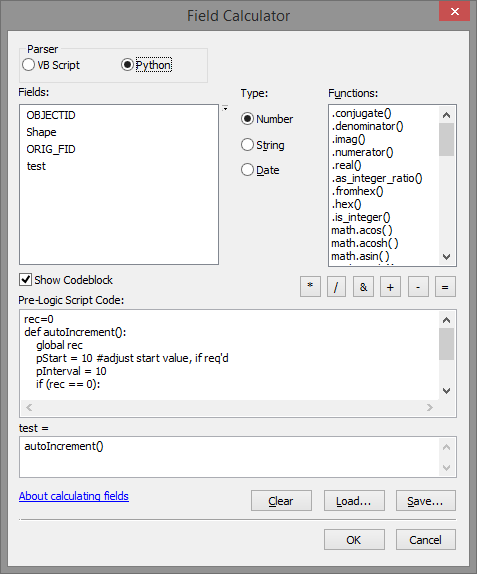# Using Increments

1651
1
Jump to solution
02-01-2016 08:09 AMOccasional Contributor II

In Arc 9.2 I was able to use the following script to calculate incremental numbers;

Static rec As Long

Dim lStart As Long

Dim lInterval As Long

Dim i As Integer

Dim iStringLength As Integer

Dim sID As String

'============================

'set the variables below

iStringLength = 7

lStart = 0001

lInterval = 1

'============================

sID = ""

If (rec = 0) Then

rec = lStart

Else

rec = rec + lInterval

End If

For i = 1 To iStringLength - Len(CStr(rec))

sID = sID & "0"

Next i

sID = sID & CStr(rec)

In Arc 10.2, am I right in saying that I can no longer use this script? I've tried the posted python example of;

rec=0

def autoIncrement():

global rec

pStart = 1 #adjust start value, if req'd

pInterval = 1 #adjust interval value, if req'd

if (rec == 0):

rec = pStart

else:

rec = rec + pInterval

return rec

This is fine, to a degree but not the results I'm truly looking for.

I'm looking to populate a 4 character field with leading zeros as well as incrementing by 10 each new record.

eg; 0010, 0020, 0030, etc up to a possible 9990.

Anyone able to help?

Thank you.

Tags (1)
1 Solution

Accepted SolutionsbyEsri Esteemed Contributor

Try this:

`rec=0def autoIncrement():    global rec    pStart = 10 #adjust start value, if req'd    pInterval = 10    if (rec == 0):        rec = pStart    else:        rec = rec + pInterval    return "{0}".format(rec).zfill(4)`1 ReplybyEsri Esteemed Contributor

Try this:

`rec=0def autoIncrement():    global rec    pStart = 10 #adjust start value, if req'd    pInterval = 10    if (rec == 0):        rec = pStart    else:        rec = rec + pInterval    return "{0}".format(rec).zfill(4)`